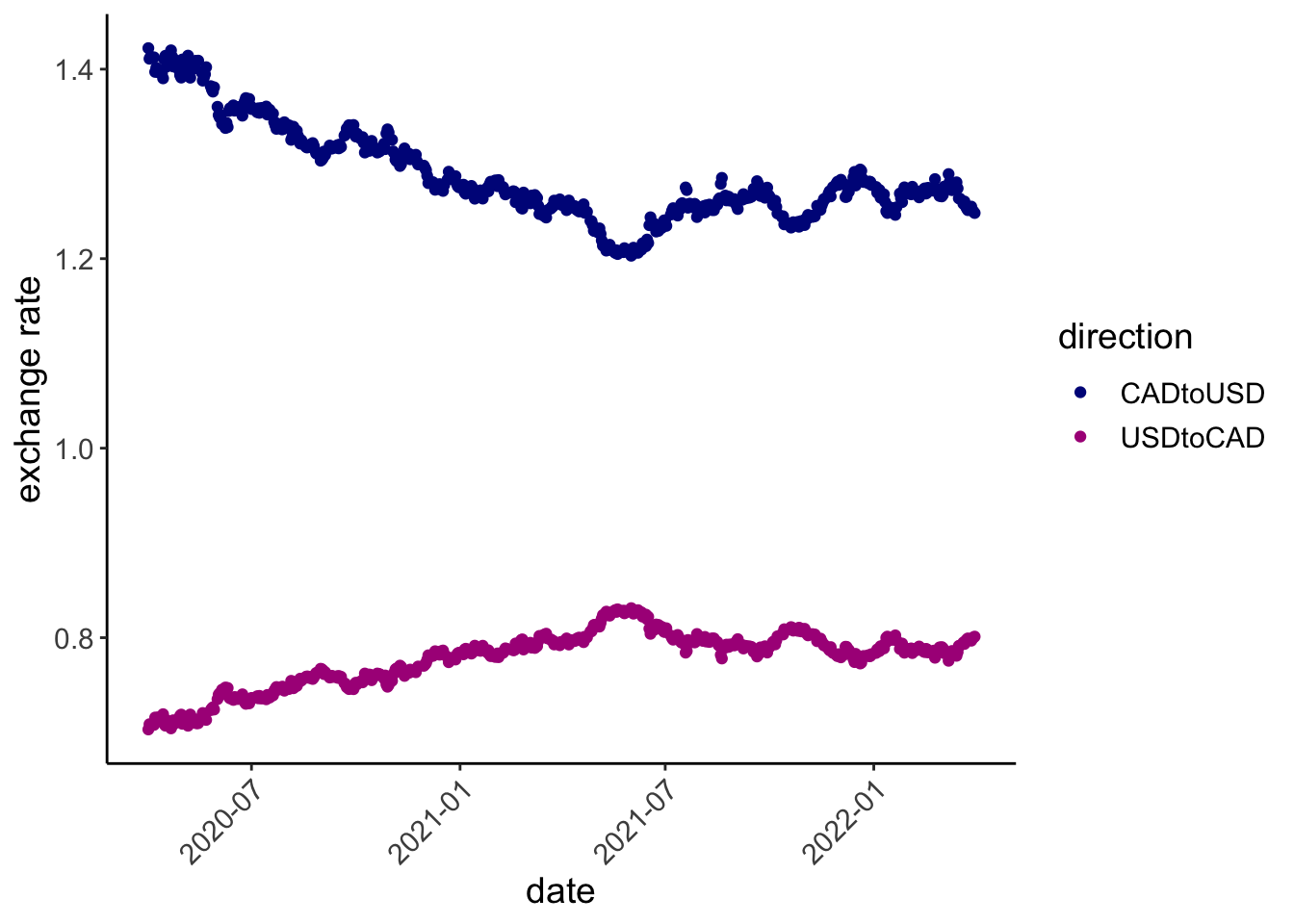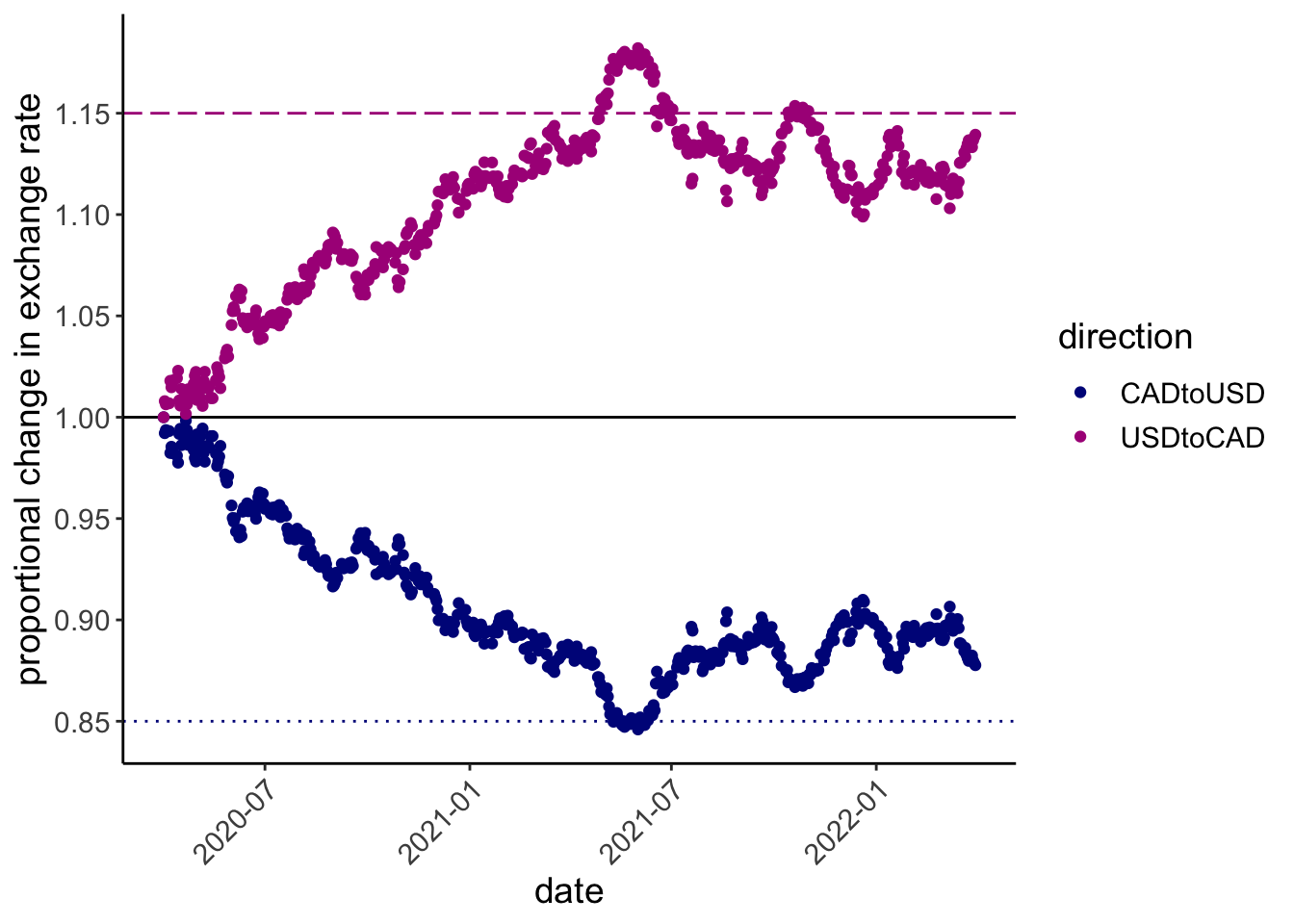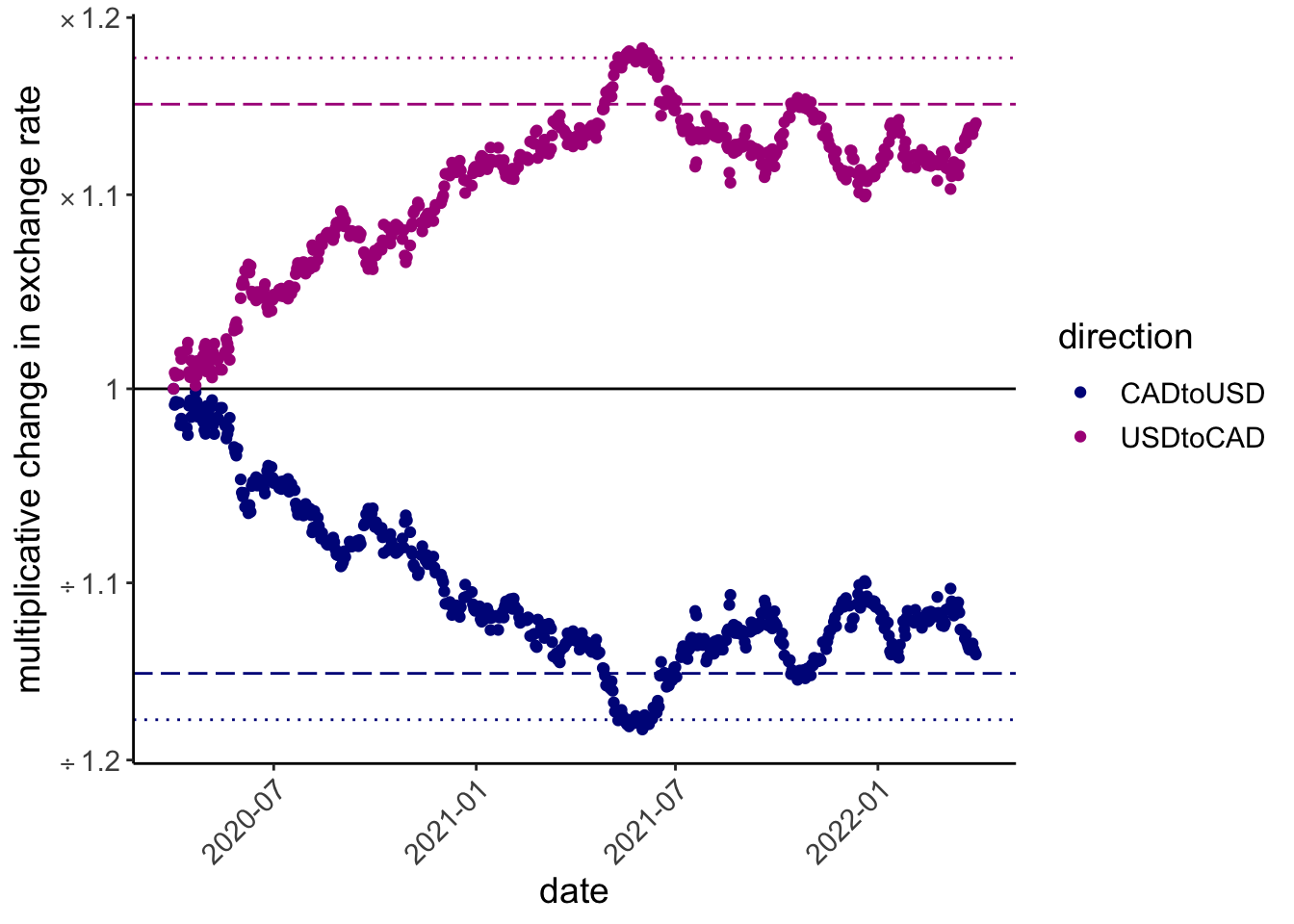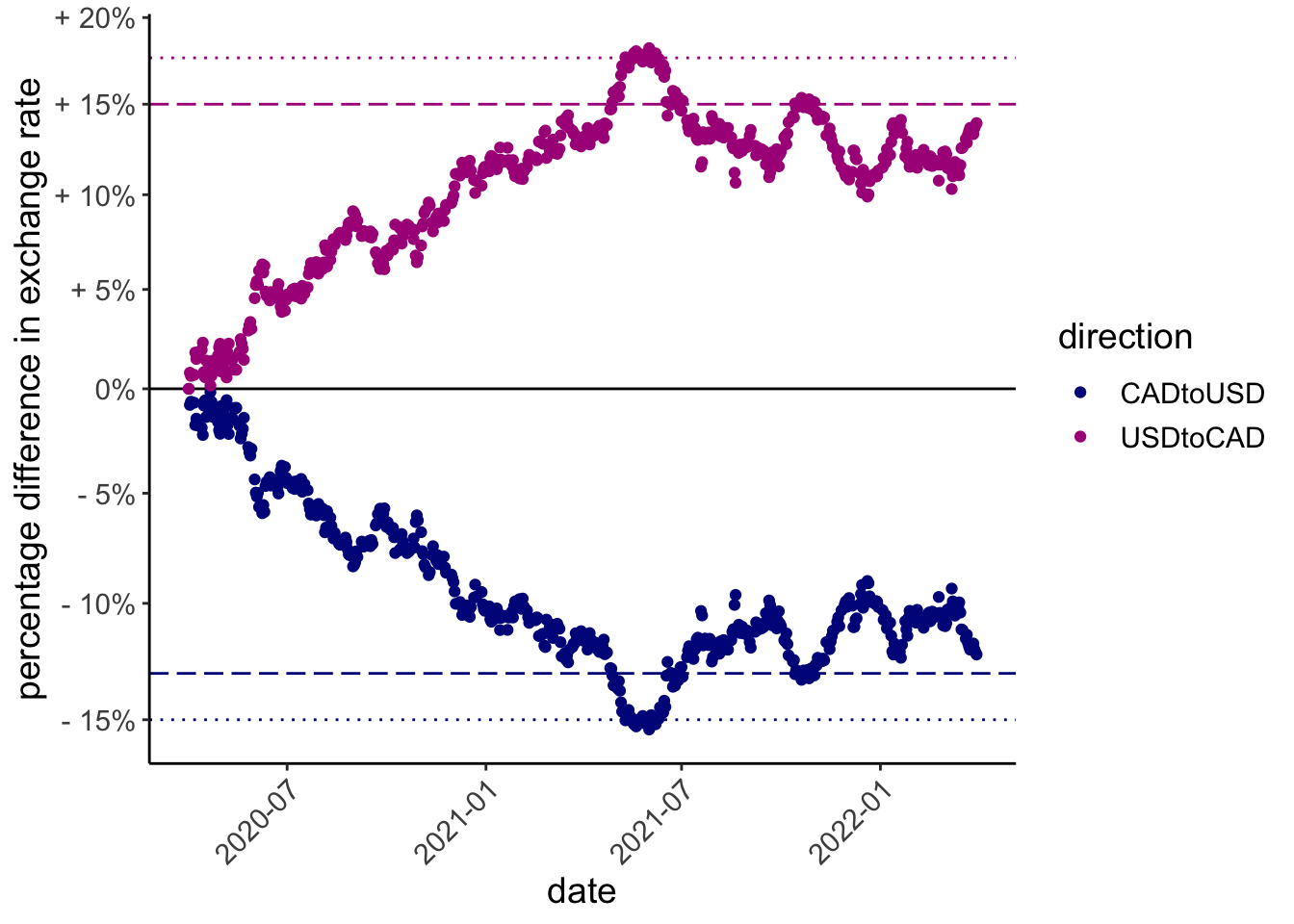Logarithmic axis scales can clearly communicate multiplicative changes; they can also confuse. ratioScales annotates logarithmic axis scales with tickmarks that denote proportional and multiplicative change simply and explicitly.

The main function in this package, scale_*_ratio(), is ggplot-friendly and works similarly to existingscale_*_* functions from ggplot2 and scales.

## Installation

You can install the development version of ratioScales from GitHub with:

# install.packages("remotes")
remotes::install_github("mikeroswell/ratioScales")

## Example

Consider exchange rates between US and Canadian dollars:


exch %>%
ggplot(aes(date, exRate, color = direction)) +
geom_point() +
scale_color_manual(values = hcl.colors(4, "Plasma")[c(1,2)]) +
labs(y = "exchange rate")Exchange rates between US and Canada during the COVID-19 pandemic

Let’s see, relative to some baseline (1 April 2020), is the Canadian dollar gaining or losing ground against the US dollar, and by how much?


exch %>%  ggplot(aes(date, exRate_scale, color = direction)) +
geom_hline(yintercept = 1, color = "black")+
geom_point() +
scale_color_manual(values = hcl.colors(4, "Plasma")[c(1,2)]) +
geom_hline(yintercept = 0.85
, color = hcl.colors(4, "Plasma"), linetype = 3) +
geom_hline(yintercept = 1.15
, color = hcl.colors(4, "Plasma"), linetype = 5) +
# FUNCTION FROM ggplot2
scale_y_continuous(breaks = seq(80, 130, 5)/100) +
labs(y = "proportional change in exchange rate")Proportional change in exchange rate from 1 April 2020 to 1 April 2022

But this is strange! Somehow the Canadian dollar weakened by a maximum of 15% before rebounding, but the US dollar strengthened by much more than 15%. Maybe not the best way to think about this?

ratioScales provides “rational” alternatives.

### divMult scale

The “divMult” scale in ratioScales shows absolute ratios, prefaced by an operator sign (e.g., × or ÷), allowing easy and accurate comparison of multiplicative changes.

exch %>%  ggplot(aes(date, exRate_scale, color = direction)) +
geom_hline(yintercept = 1, color = "black")+
# times and divided by 1.15; longdash
geom_hline(yintercept = 1/1.15
, color = hcl.colors(4, "Plasma")
, linetype = 5) +
geom_hline(yintercept = 1.15
, color = hcl.colors(4, "Plasma")
, linetype = 5) +
# times and divided by 0.85; dotted
geom_hline(yintercept = 1/0.85
, color = hcl.colors(4, "Plasma")
, linetype = 3) +
geom_hline(yintercept = 0.85
, color = hcl.colors(4, "Plasma")
, linetype = 3) +
geom_point() +
scale_color_manual(values = hcl.colors(4, "Plasma")[c(1,2)]) +
# FUNCTION FROM ratioScales
scale_y_ratio(tickVal = "divMult", n = 12, nmin = 12, slashStar = FALSE) +
labs(y = "multiplicative change in exchange rate")exchange rate changes on the divMult scale

### percDiff

Prefer percentage differences? You can do this in a principled fashion using scale_y_ratio(tickVal = "percDiff").

exch %>%  ggplot(aes(date, exRate_scale, color = direction)) +
geom_hline(yintercept = 1, color = "black")+
# times and divided by 1.15; longdash
geom_hline(yintercept = 1/1.15
, color = hcl.colors(4, "Plasma")
, linetype = 5) +
geom_hline(yintercept = 1.15
, color = hcl.colors(4, "Plasma")
, linetype = 5) +
# times and divided by 0.85; dotted
geom_hline(yintercept = 1/0.85
, color = hcl.colors(4, "Plasma")
, linetype = 3) +
geom_hline(yintercept = 0.85
, color = hcl.colors(4, "Plasma")
, linetype = 3) +
geom_point() +
scale_color_manual(values = hcl.colors(4, "Plasma")[c(1,2)]) +
# FUNCTION FROM ratioScales
scale_y_ratio(tickVal = "percDiff") +
labs(y = "percentage difference in exchange rate")caption explaining the reference lines

This preserves the true ratio-based differences on the visual plot, but the values on the guide do not correspond simply to ratio differences (and are not symmetric, see plot).

### centiNels

We can also make a plot that will often be very similar to the percDiff plot, but which uses numbers that are better for quantitative analysis.